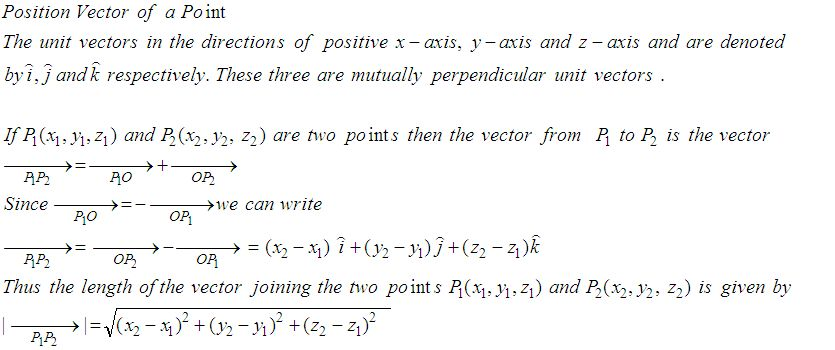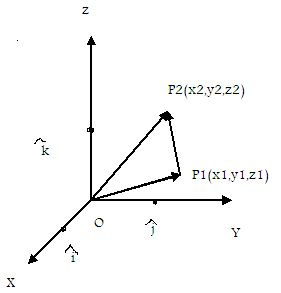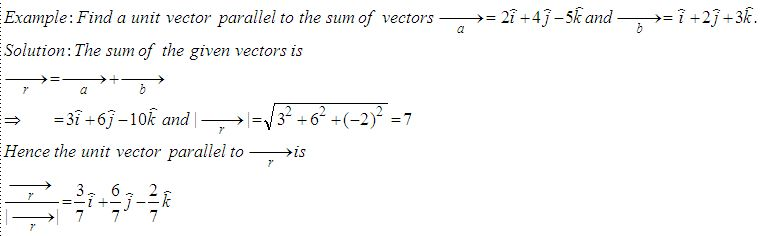Name: ___________________Date:___________________

 Email us to get an instant 20% discount on highly effective K-12 Math & English kwizNET Programs!

High School Mathematics - 25.9 Position Vector of a PointDirections: Answer the following.Name: ___________________Date:___________________

High School Mathematics - 25.9 Position Vector of a Point

 Q 1: Find the vector joining the initial point P(-4,2) and terminal point Q(0,-4) 8i-6j4i-6j4i+6j Q 2: Find a unit vector in the direction from P(3,2) towards Q(5,6).4i+5i/Ö5 + 2j/Ö52i+3 Q 3: Find a unit vector parallel to the sum of vectors a = 2i+4j-5k, b = i+2j+3k8i+4j-3k3/7i + 6/7j - 2/7 k2-i8 Q 4: If P = 2,4,7 and P2 = (-4, -1, 5) then find vector P1P2.-6i+5j6i+5j+3k-6i-5j-2k Q 5: If P = 2,4,7 and P2 = (-4, -1, 5) then find the magnitude of vector P1P2.3i+6jÖ656i+5j Q 6: Find the vector with initial point P(6,-2) and terminal point Q(4,-8).6i-9j-2i+10j8i+2j Q 7: Find the vector in the direction of the vector -i+2j+2k that has magnitude 7.7(-i+2j+2k)/3i-j+k-3i+2j Q 8: Find the unit vector in the direction of P(1,2,3) towards Q(4,5,6).6i+5j+2k(i+j+k)/Ö38j-2k Question 9: This question is available to subscribers only! Question 10: This question is available to subscribers only!